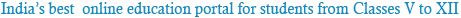### NCERT Solutions for Class 11th Chemistry Chapter 1: Some basic Concepts of Chemistry

Question 1: Find out the value of molecular weight of the given compounds:
(i) CH4 (ii)H2O (iii)CO2
(i)CH4 : Molecular weight of methane, CH4
= (1 x Atomic weight of carbon) + (4 x Atomic weight of hydrogen)
= [1(12.011 u) +4 (1.008u)]
= 12.011u + 4.032 u
= 16.043 u
(ii) H2O :
Molecular weight of water, H2O
= (2 x Atomic weight of hydrogen) + (1 x Atomic weight of oxygen)
= [2(1.0084) + 1(16.00 u)]
= 2.016 u +16.00 u
= 18.016u
So approximately
= 18.02 u
(iii) CO2 :
= Molecular weight of carbon dioxide, CO2
= (1 x Atomic weight of carbon) + (2 x Atomic weight of oxygen)
= [1(12.011 u) + 2(16.00 u)]
= 12.011 u +32.00 u
= 44.011 /u /
So approximately
= 44.01u

Question 2: Sodium Sulphate (Na2SO4) has various elements, find out the mass percentage of each element.
Now for Na2SO4. Molar mass of Na2SO4
= [(2 x 23.0) + (32.066) + 4(16.00)] =142.066 g
Formula to calculate mass percent of an element =
Mass of that element in the compound Molar mass of the compound × 100
Therefore, Mass percent of the sodium element:
= 46.0g142.066g×100 = 32.379 =32.4%
Mass percent of the sulphur element:
=32.066g142.066g×100 = 22.57 =22.6%
Mass percent of the oxygen element:
= 64.0g142.066g×100 =45.049 =45.05%

Question 3:Find out the empirical formula of an oxide of iron having 69.9% Fe and 30.1% O2 by mass.
Percent of Fe by mass = 69.9 % [As given above]
Percent of O2 by mass = 30.1 % [As given above]
Relative moles of Fe in iron oxide:
= percent of iron by mass Atomic mass of iron
= 69.955.85 = 1.25
Relative moles of O in iron oxide:
= percent of oxygen by mass Atomic mass of oxygen
= 30.116.00 = 1.88
Simplest molar ratio of Fe to O:
= 1.25: 1.88
= 1: 1.5 ≈ 2: 3
Therefore, empirical formula of iron oxide is Fe2O3.

Question 4:Find out the amount of CO2 that can be produced when
(i) 1 mole carbon is burnt in air.
(ii) 1 mole carbon is burnt in 16 g of O2.
(iii) 2 moles carbon are burnt in 16 g O2.
(i) 1 mole of carbon is burnt in air.
C+O2→CO2
1 mole of carbon reacts with 1 mole of O2 to form one mole of CO2.
Amount of CO2 produced = 44 g
(ii) 1 mole of carbon is burnt in 16 g of O2.
1 mole of carbon burnt in 32 grams of O2 it forms 44 grams of CO2.
Therefore, 16 grams of O2 will form 44×1632
= 22 grams of CO2
(iii) 2 moles of carbon are burnt in 16 g of O2.
If 1 mole of carbon are burnt in 16grams of O2 it forms 22 grams of CO2
Therefore, if 2 moles of carbon are burnt it will form
= 2×221
= 44g of CO2

Question 5:Find out the mass of CH3COONa(sodium acetate) required to make 500 mL of 0.375 molar aqueous solution. Molar mass of CH3COONa is 82.0245gmol−1
0.375 Maqueous solution of CH3COONa
= 1000 mL of solution containing 0.375 moles of CH3COONa
Therefore, no. of moles of CH3COONa in 500 mL
= 0.3751000×1000
= 0.1875 mole
Molar mass of sodium acetate = 82.0245gmol−1
Therefore, mass that is required of CH3COONa
= (82.0245gmol−1)(0.1875mole)
= 15.38 gram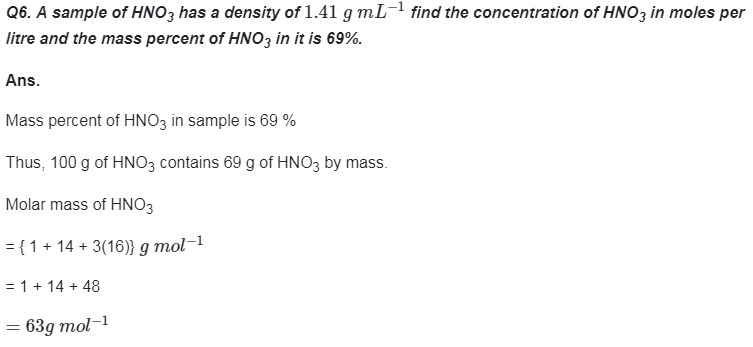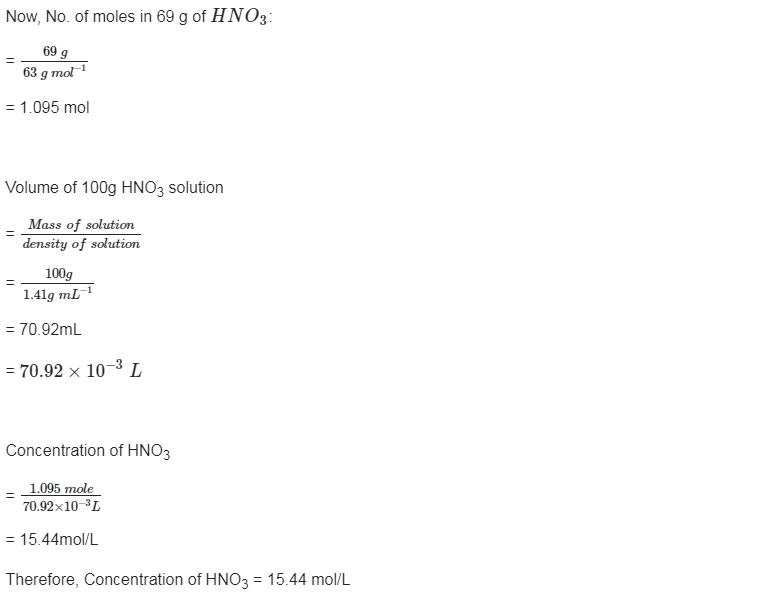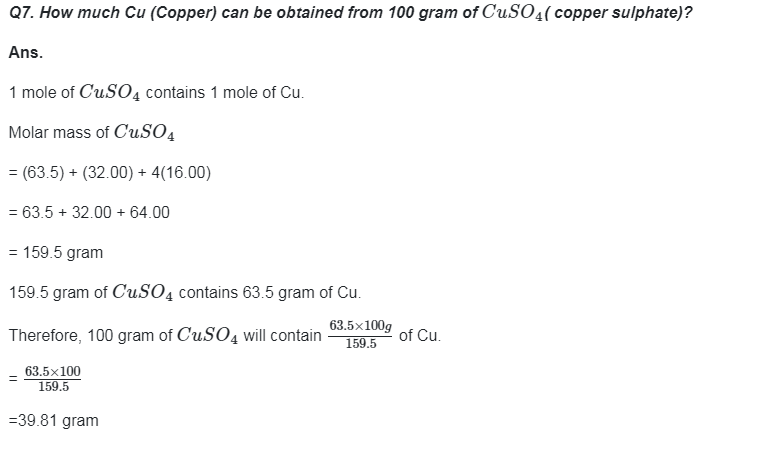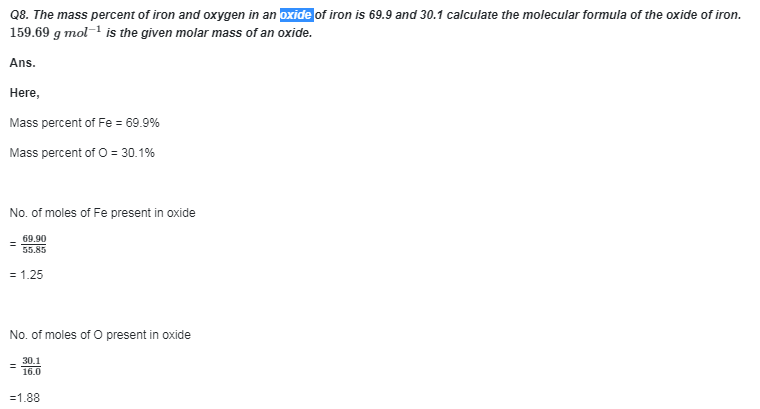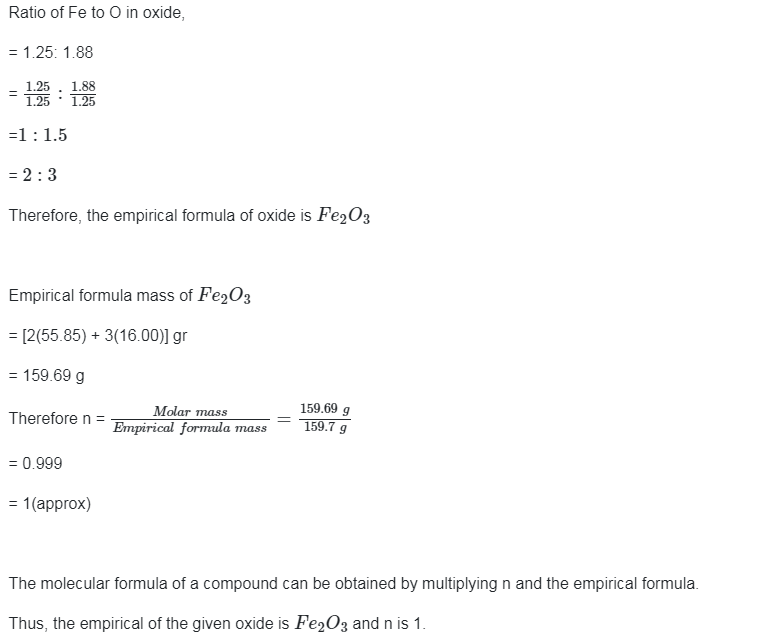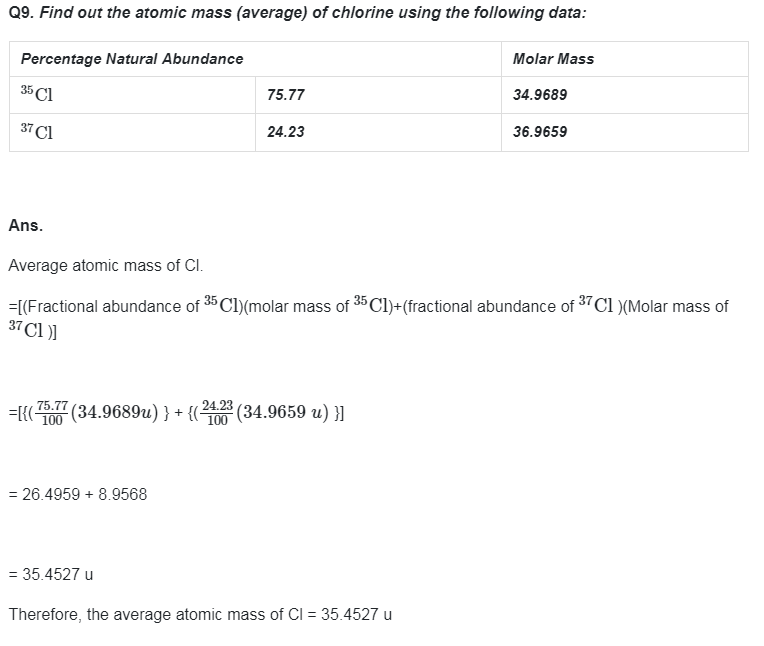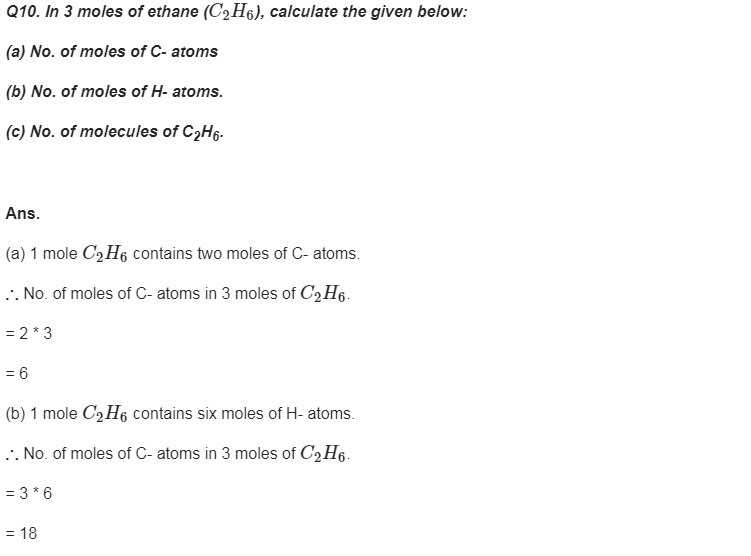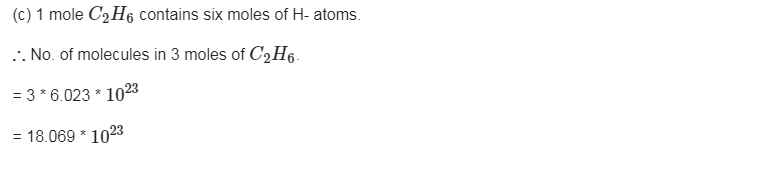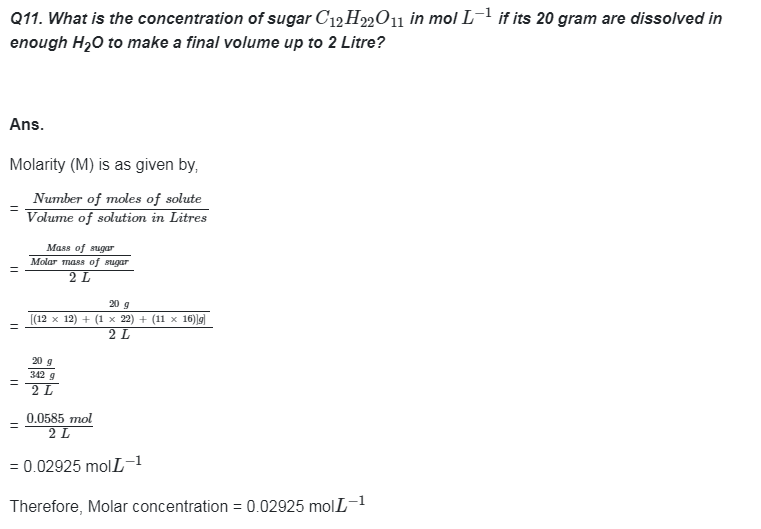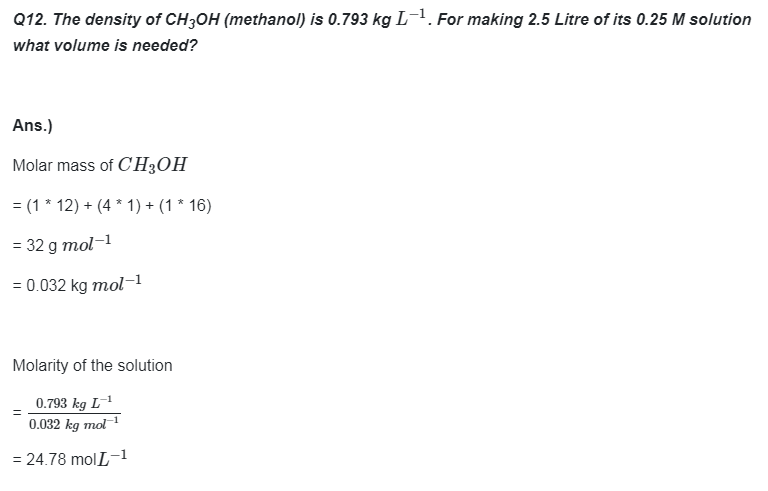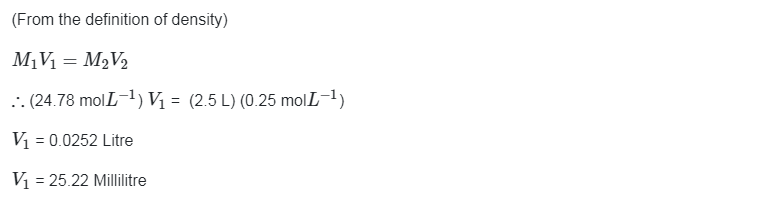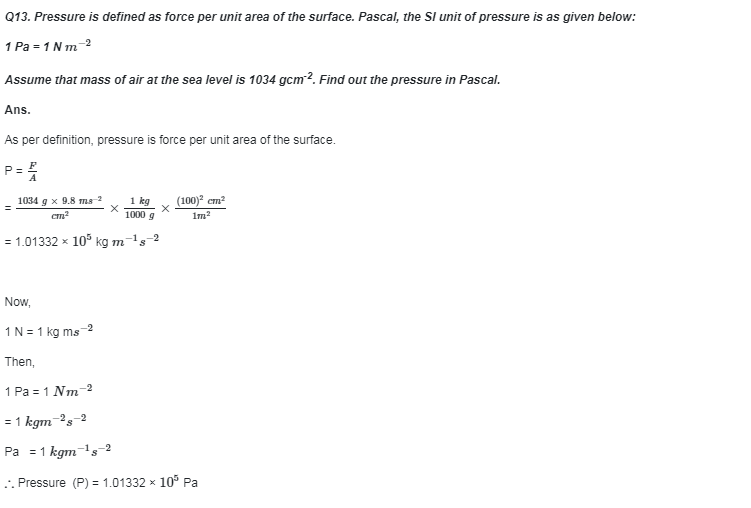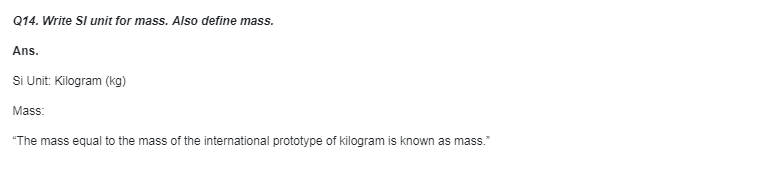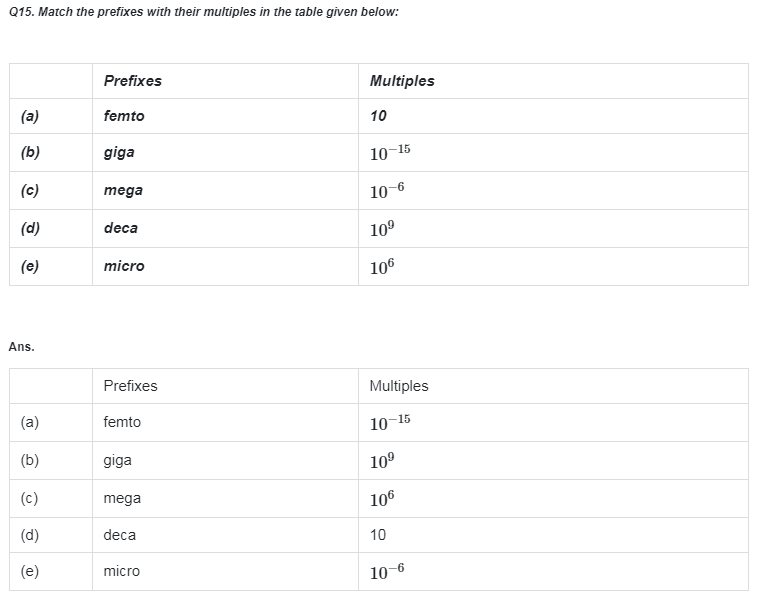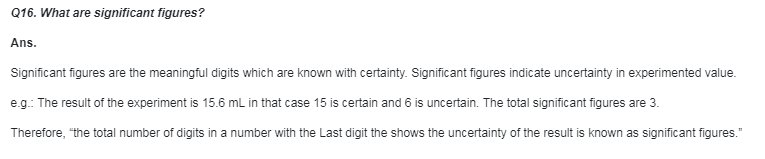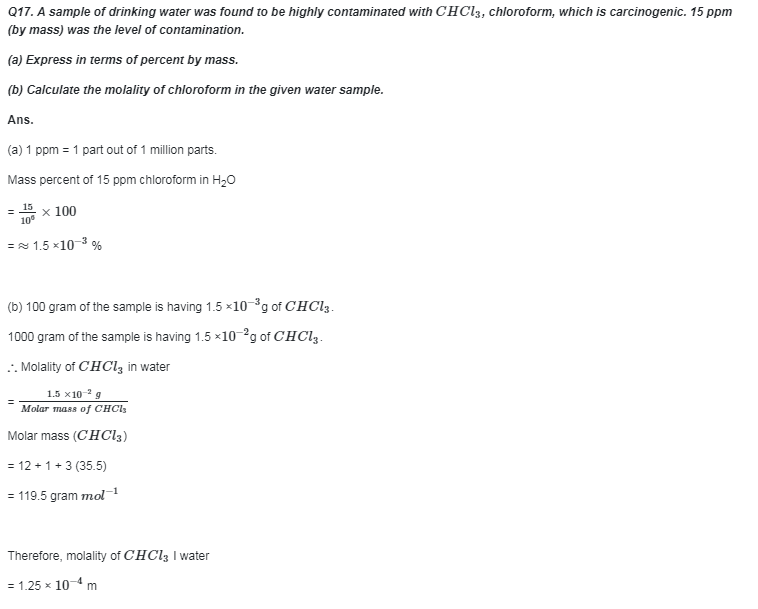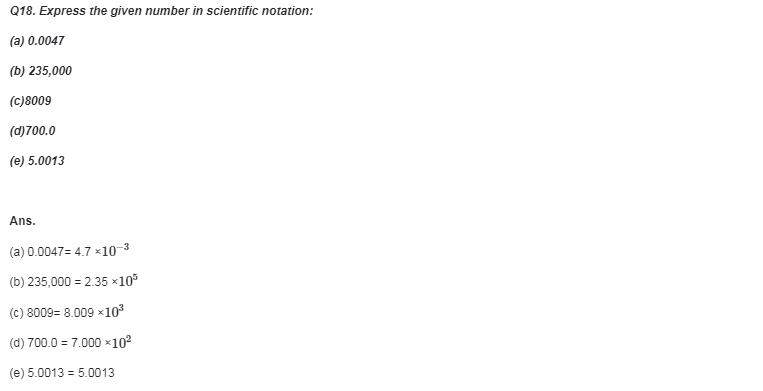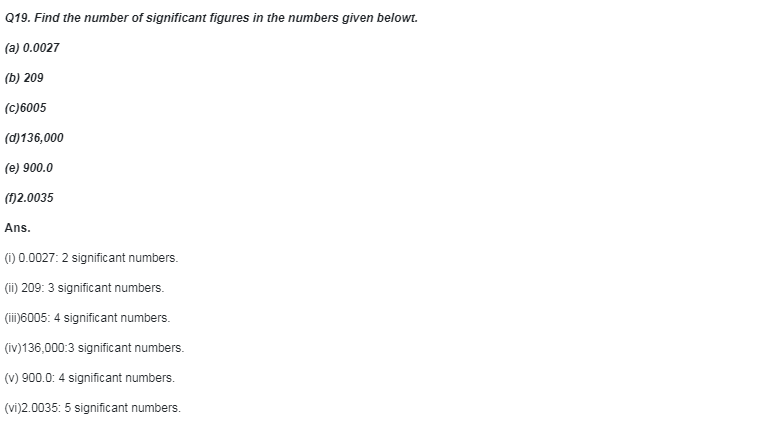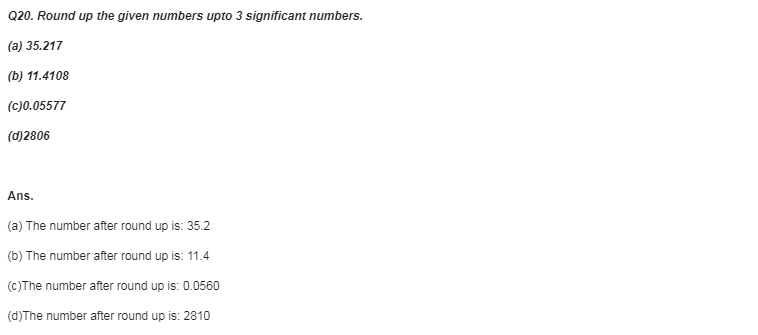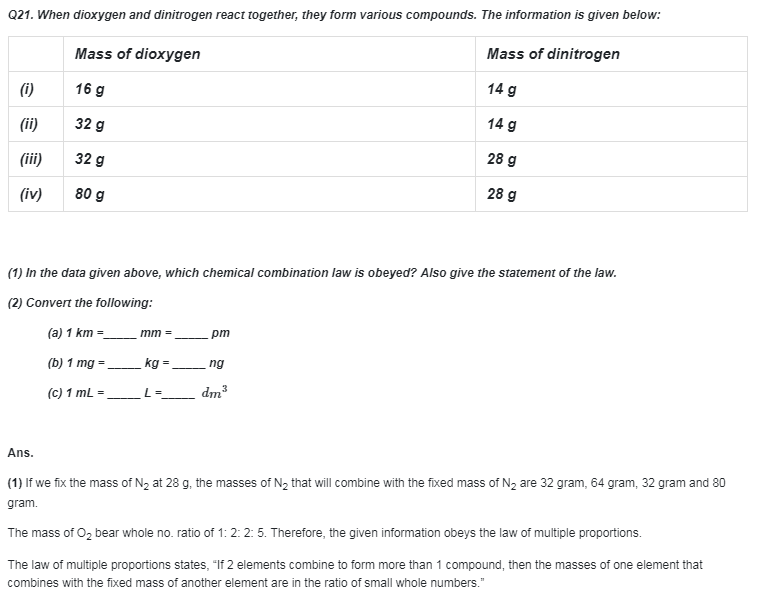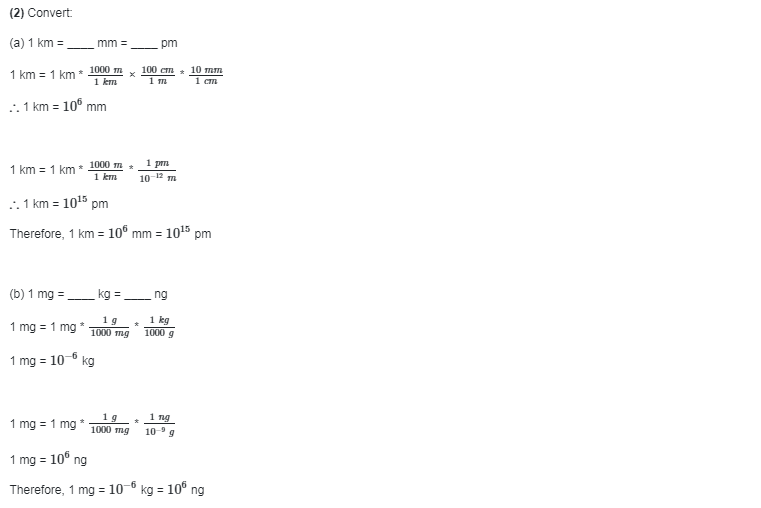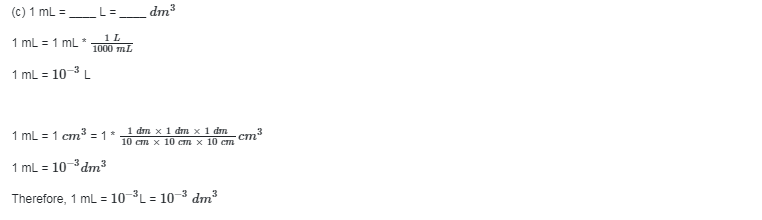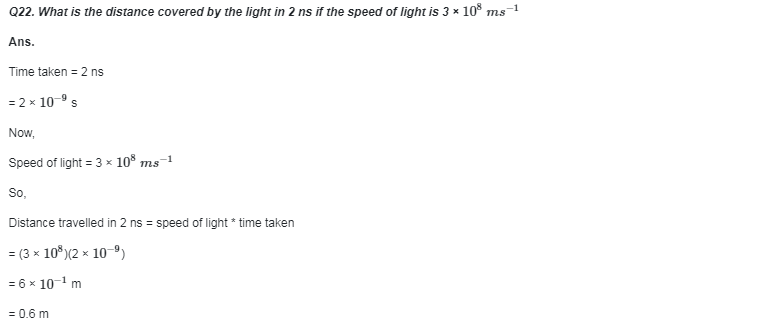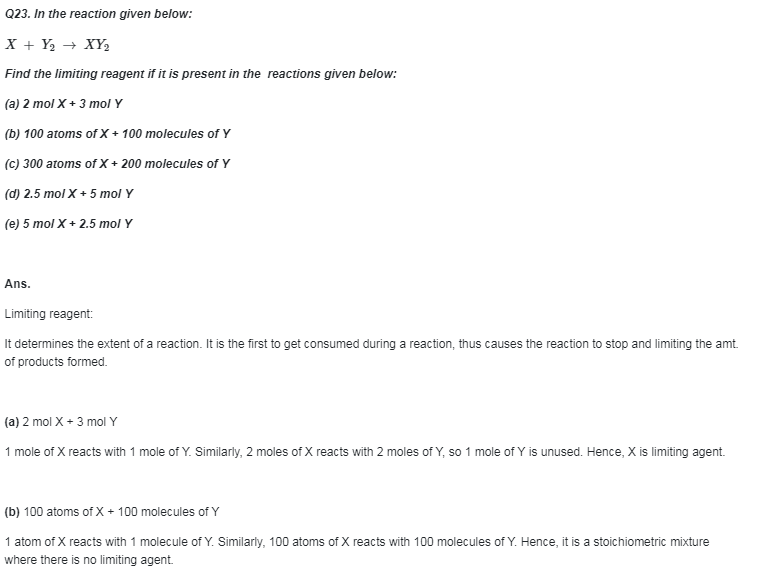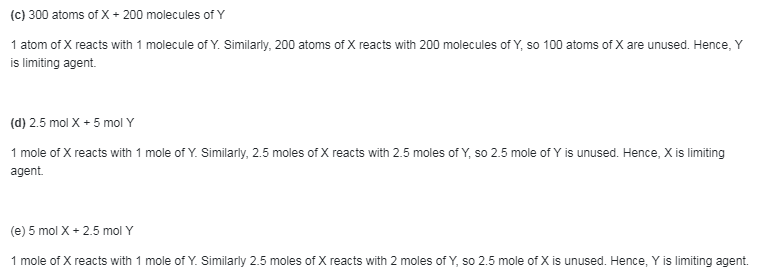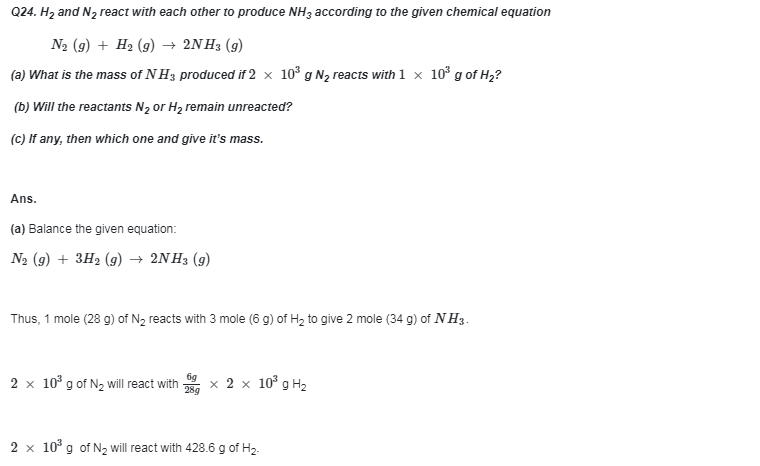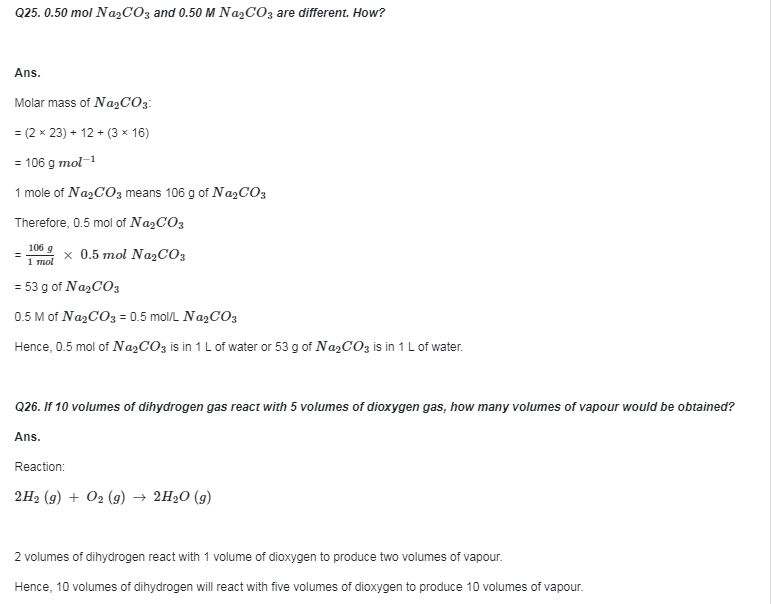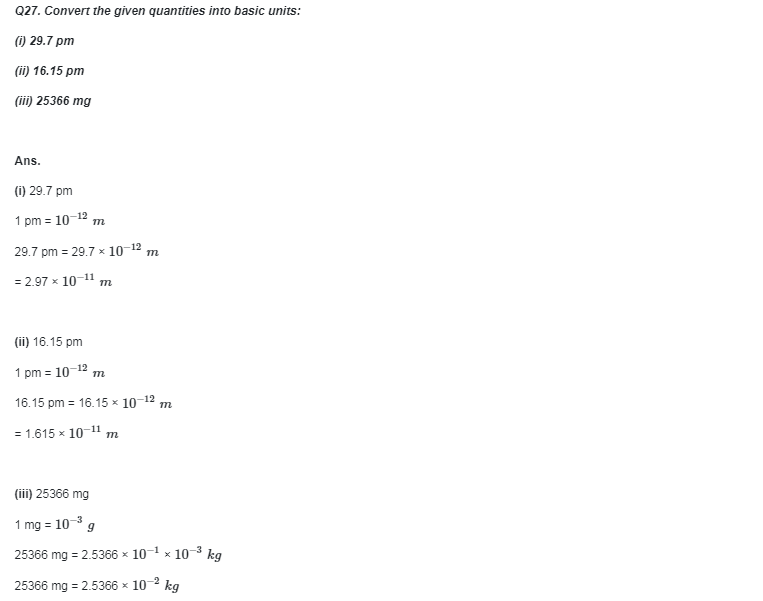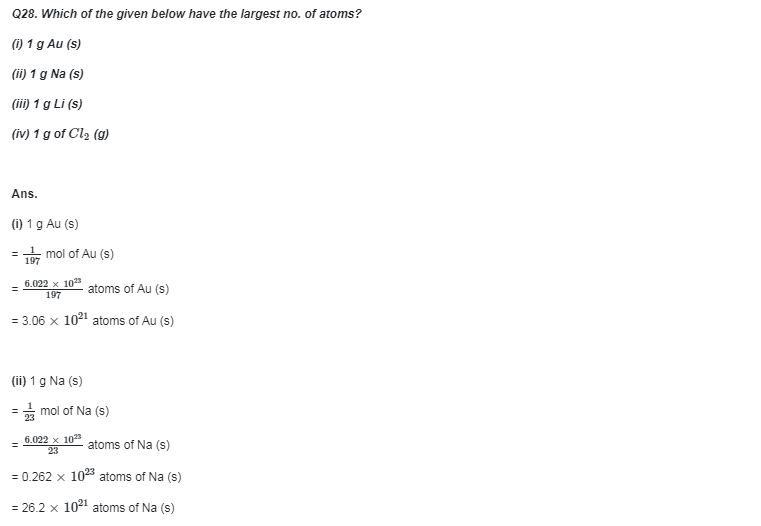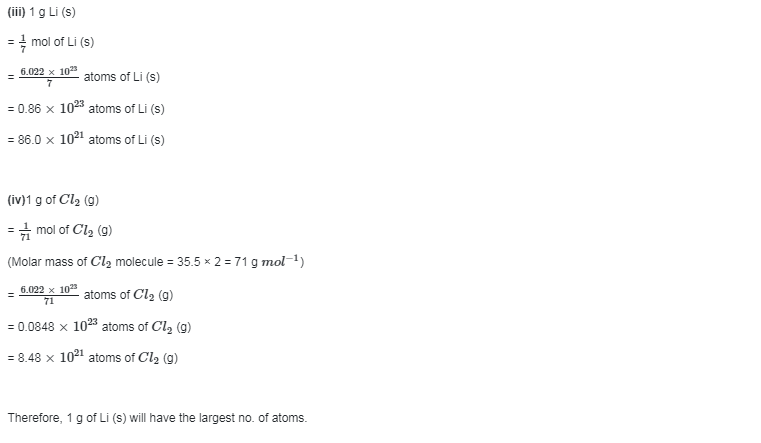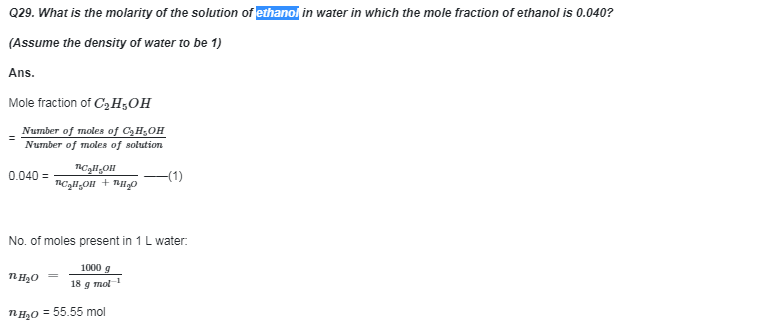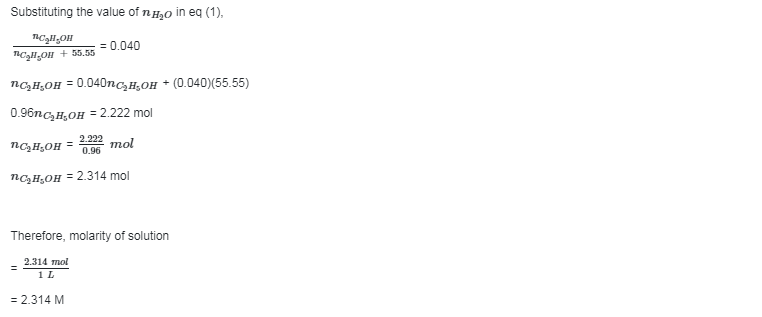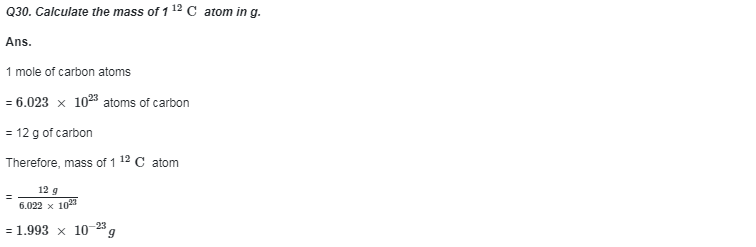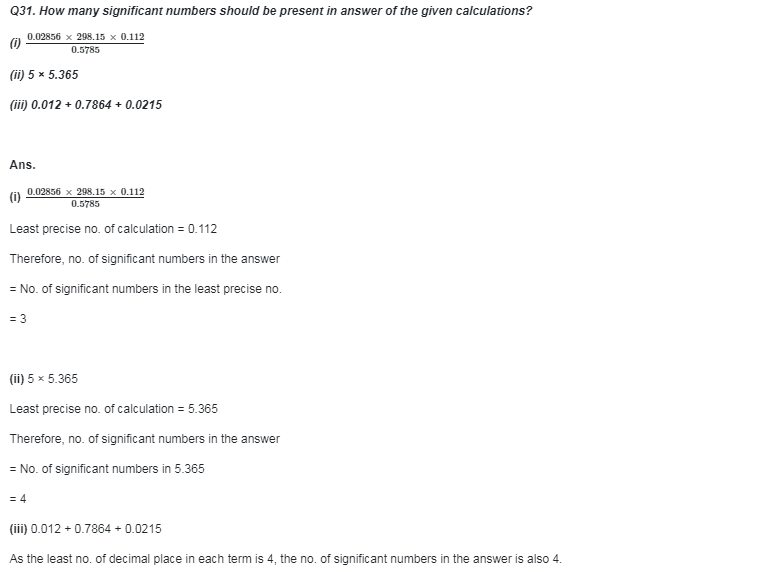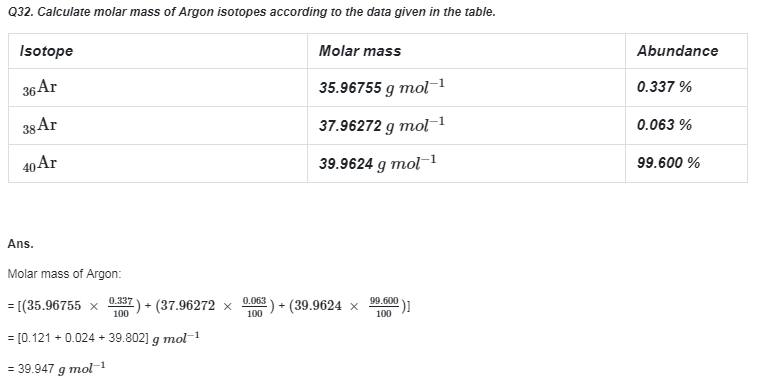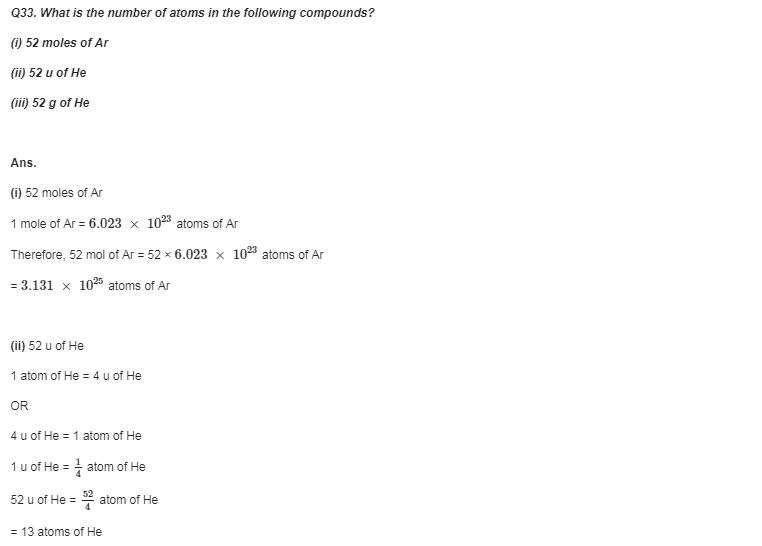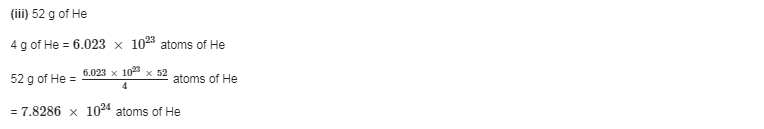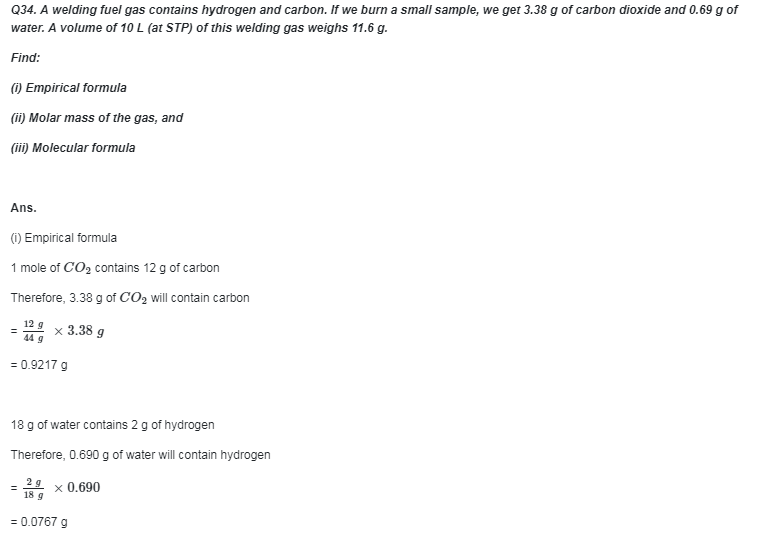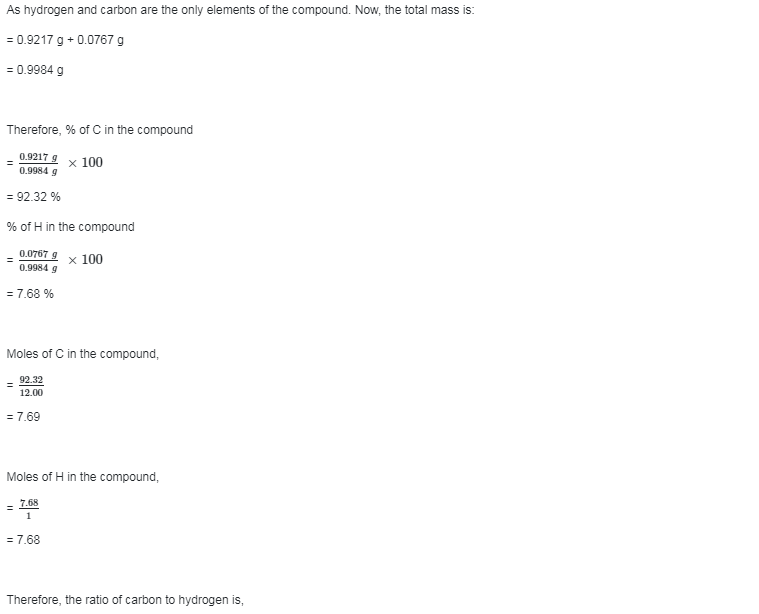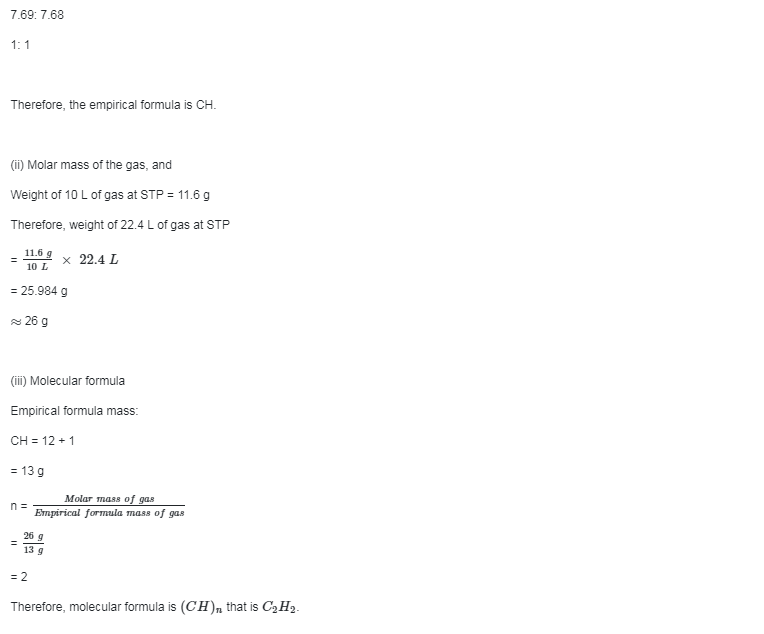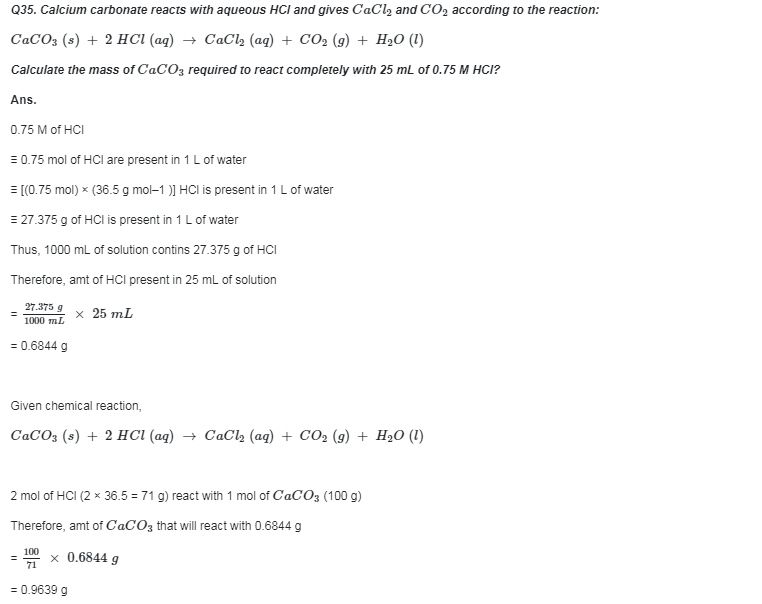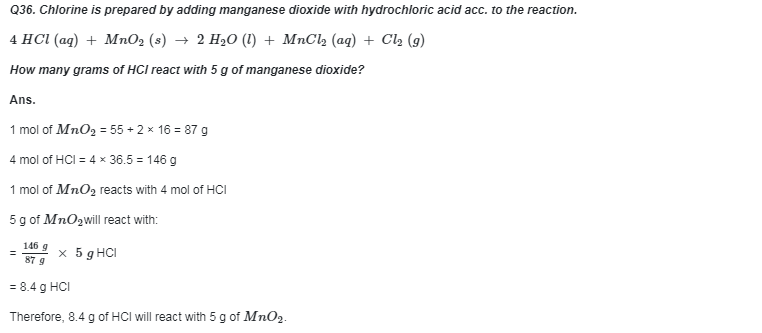Powered by: iVerticals Web Technology Pvt Ltd